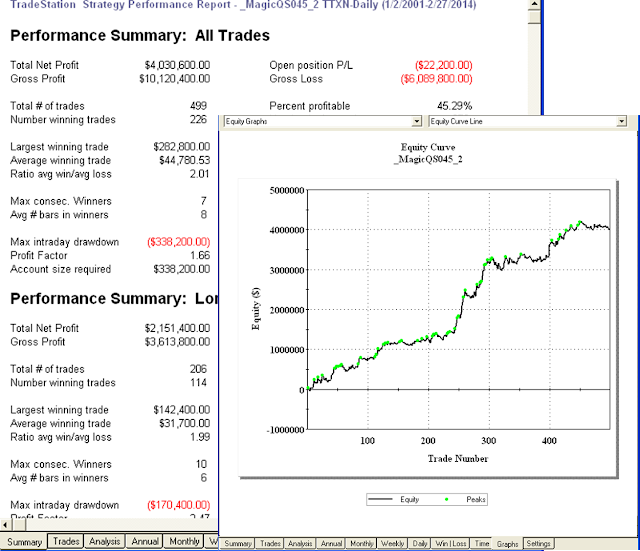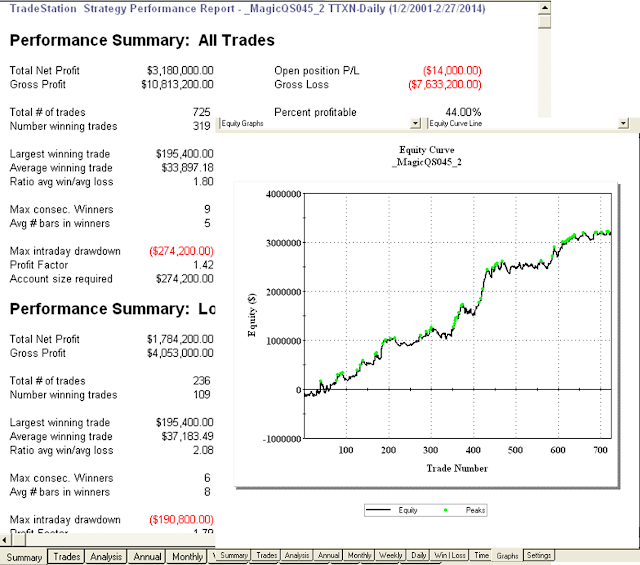## 2014年3月3日 星期一

### DMI趨向指標的交易模型  (程式碼)

1.+DI為上漲方向指標，+DI值愈高，代表漲勢愈明確且強烈；-DI為下跌方向指標，-DI值愈高時，代表跌勢愈明確且乏力。

DMI 測試inputs: XL1(10),XS1(10),XL2(10),XS2(1),NL1(10),NS1(8),NL2(10),NS2(15),SFT_L1(10),SFT_S1(6),SFT_L2(100),SFT_S2(10) ;

inputs: NBar_LE(9),Frac_LE(1.74),TRLen_L(2),Ratio_TL(2.38),TRPct_L(60),NBar_LM(9),Frac_LMM(1.3),NBar_LX(12),Bar_L1(3) ;

inputs: NBar_SE(5),Frac_SE(1.2),TRLen_S(40),Ratio_TS(1.2),TRPct_S(60),NBar_SM(6),Frac_SMM(1.2),NBar_SX(10),Bar_S1(3) ;

Var:LE_ATR(0),TL_ATR(0),LMM_ATR(0),SE_ATR(0),TS_ATR(0),SMM_ATR(0) ;
Var:VarL1(0),VarL2(0),VarL3(0),VarL4(0),VarS1(0),VarS2(0),VarS3(0),VarS4(0),Cond_LE(false),Cond_SE(false);

LE_ATR = Average(TrueRange, NBar_LE);
LMM_ATR = Average(TrueRange, NBar_LM);
TL_ATR = Average(TrueRange, TRLen_L);

UBuy = Low + Frac_LE * LE_ATR;
USell = Low ;

VarS1 = DMI(NS1)[SFT_S1];
VarS2 = DMI(NS2);

Cond_LE = true;
Cond_SE = VarS1 >= VarS2;

If Cond_LE then begin
end;

If Cond_SE then begin
Sell next bar at USell stop;
end;

If MarketPosition > 0 then begin
If BarsSinceEntry = 0 then begin
BuyStop = EntryPrice - Frac_LMM * LMM_ATR;
Trigger_TL = false;
end;

If Close - EntryPrice > Ratio_TL * TL_ATR then  Trigger_TL = true;

If Trigger_TL then begin
NewBuyStop = EntryPrice + TRPct_L * (Close - EntryPrice)/100.;